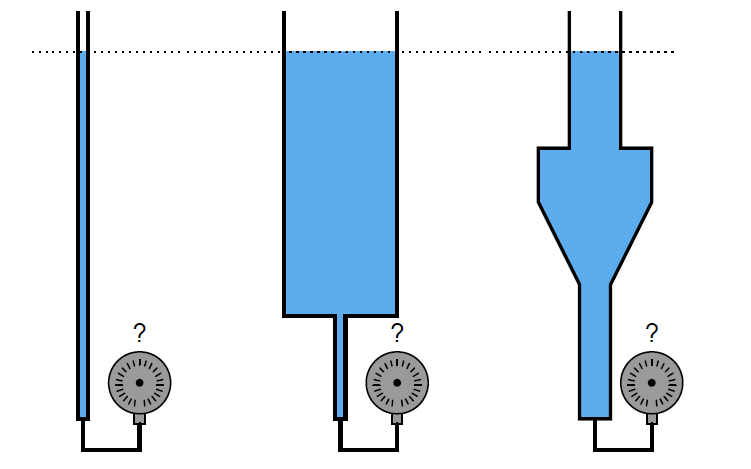# Which Tube has most Hydrostatic Pressure?

Which of these tubes will generate the most hydrostatic pressure, assuming they all contain the same type of liquid at precisely the same (vertical) height?

## Hydrostatic PressureThis is a “trick” question: they all generate the exact same amount of hydrostatic pressure.

The principle at work here is the relationship between vertical height and hydrostatic pressure. Cross-sectional area of the liquid column is irrelevant! Only column height, liquid density, and the gravitational pull of the Earth matter when calculating hydrostatic pressure.

Since all three of these variables are precisely the same in this scenario, the hydrostatic pressures must likewise be precisely the same.

Credits: Tony R. Kuphaldt

1.